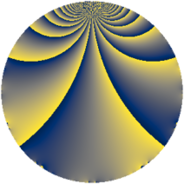# Properties

 Label 2001.2.dLevel $2001$ Weight $2$ Character orbit 2001.d Rep. character $\chi_{2001}(1103,\cdot)$ Character field $\Q$ Dimension $224$ Sturm bound $480$

# Related objects

## Defining parameters

 Level: $$N$$ $$=$$ $$2001 = 3 \cdot 23 \cdot 29$$ Weight: $$k$$ $$=$$ $$2$$ Character orbit: $$[\chi]$$ $$=$$ 2001.d (of order $$2$$ and degree $$1$$) Character conductor: $$\operatorname{cond}(\chi)$$ $$=$$ $$69$$ Character field: $$\Q$$ Sturm bound: $$480$$

## Dimensions

The following table gives the dimensions of various subspaces of $$M_{2}(2001, [\chi])$$.

Total New Old
Modular forms 244 224 20
Cusp forms 236 224 12
Eisenstein series 8 0 8

## Trace form

 $$224q - 4q^{3} - 216q^{4} + 4q^{9} + O(q^{10})$$ $$224q - 4q^{3} - 216q^{4} + 4q^{9} + 16q^{12} + 216q^{16} - 32q^{18} + 264q^{25} - 16q^{27} + 8q^{31} + 24q^{36} + 32q^{39} + 12q^{46} - 132q^{48} - 240q^{49} - 40q^{52} - 52q^{54} - 72q^{55} - 152q^{64} - 12q^{69} + 40q^{70} + 92q^{72} - 48q^{73} - 12q^{75} + 104q^{78} - 36q^{81} - 120q^{82} - 48q^{85} + 16q^{93} + 168q^{94} - 24q^{96} + O(q^{100})$$

## Decomposition of $$S_{2}^{\mathrm{new}}(2001, [\chi])$$ into newform subspaces

The newforms in this space have not yet been added to the LMFDB.

## Decomposition of $$S_{2}^{\mathrm{old}}(2001, [\chi])$$ into lower level spaces

$$S_{2}^{\mathrm{old}}(2001, [\chi]) \cong$$ $$S_{2}^{\mathrm{new}}(69, [\chi])$$$$^{\oplus 2}$$